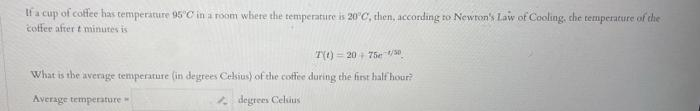# (Solved): If a cup of colfee has temperiture $$95^{\circ} \mathrm{C}$$ in a room where the temperature is \ ...If a cup of colfee has temperiture $$95^{\circ} \mathrm{C}$$ in a room where the temperature is $$20^{\circ} \mathrm{C}$$, then, accordang to Newton's law of Cooling, the temperature of the c?otfee after tominutes is $T(t)=20+75 e^{-t / 30}$ What is the average temperature (in degrees Celsias) of the coffee during the first half hour? Average temperature = degrtees Celuas

We have an Answer from Expert